CADinTools - Macros for CorelDraw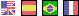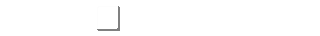# CURVE EDITION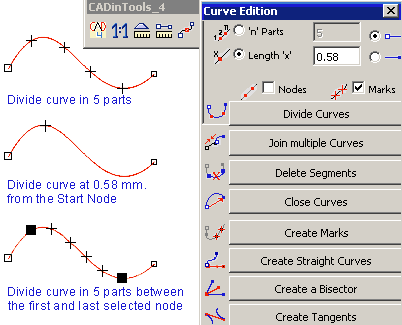•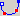Divide curves (Selection of one or more curves) :
•'n' Parts: Divide a curve in 2 or more parts.
•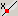Length 'x': Divide a curve at 'x' length from the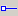start point or the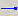end point.
• Options: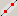Divide with Nodes,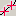Divide with Marks.
• Note: If a node range is selected, the division is applied between the first and last node selected. If the curve has more than one subpath, subpaths are treated independently.
•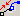Join multiple curves :  Join two or more curves (only if their ending nodes are overlapped)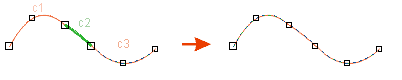•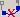Delete segments : Delete all segments between the first and last selected node.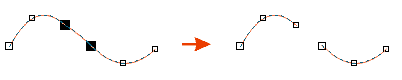•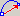Close curves : Close all selected curves.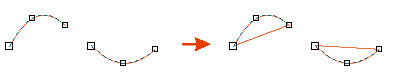•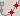Create Marks : Create a Mark on all the nodes of all selected curves. Select a node range to Mark only the selected nodes.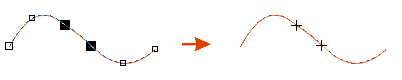•Create straight curves : Create a straight curve with the same length and number of nodes as the original curve.•Create a Bisector : Create a Bisector between 2 selected curves. Create a Bisector from a selected node.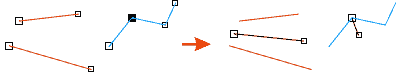•Create Tangents : Create all Tangents between two selected curves.Note1: The measure unit is the actual unit of the active document.
Note2: You can use formulas in the text boxes ("See the Calculator Tool")